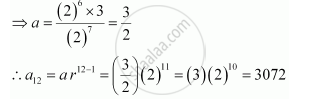CBSE (Arts) Class 11CBSE
Share
Notifications

View all notifications
Books Shortlist
Your shortlist is empty

# Find the 12th Term of a G.P. Whose 8th Term is 192 and the Common Ratio is 2. - CBSE (Arts) Class 11 - Mathematics

Login
Create free account

Forgot password?
ConceptGeometric Progression (G. P.)

#### Question

Find the 12th term of a G.P. whose 8th term is 192 and the common ratio is 2.

#### Solution

Common ratio, r = 2

Let a be the first term of the G.P.

∴ a8 = ar 8–1 = ar7

⇒ ar7 = 192

a(2)7 = 192

a(2)7 = (2)6 (3)Is there an error in this question or solution?

#### APPEARS IN

NCERT Solution for Mathematics Textbook for Class 11 (2018 to Current)
Chapter 9: Sequences and Series
Q: 2 | Page no. 192
Solution Find the 12th Term of a G.P. Whose 8th Term is 192 and the Common Ratio is 2. Concept: Geometric Progression (G. P.).
S